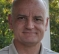## Filtering of amplitude spectrumStarted by 6 years ago7 replieslatest reply 6 years ago321 views

Hello,

I want to perform moving average on the amplitude spectrum of a speech signal frame (abs(fft(sig))) in the particular frequency range (e.g. 4-8kHz, Fs=16kHz). In other words, I want to smooth the amplitude spectrum in the desired frequency range. How can it be performed?

[ - ]Do you really want to perform a moving average on the amplitude, as opposed to performing a moving average on the signal itself?

If the answer is yes, then just multiply the signal by a sinc signal of appropriate width, point-by-point, before taking the FFT.

If the answer is "on reflection, I'm not sure if I know what I'm doing" then tell us the result you're trying to achieve (low pass filtering of your signal, better spectral display, whatever), and we'll suggest methods.

[ - ]Here I am displaying what I am trying to achieve.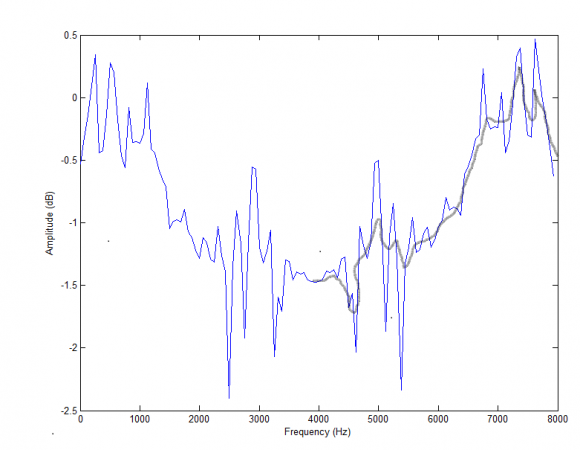Blue plot shows the magnitude spectrum of a speech frame with Fs=8kHz after zero insertion in time domain, a kind of upsampling. I want to smooth the spectrum in the range 4-8kHz (as shown by grey plot). The grey plot is randomly drawn for an illustration. Not sure if its possible to do it but I want to try some experiments this way.

[ - ]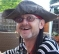In that case, as has been suggested, you need to apply a moving average filter to the data points you are collecting (assuming that the source of the curve you have illustrated is indeed from discrete data points). Sometimes, it is useful to smooth frequency response data on a log scale, which them results in fractional octave smoothing, which you may wish to google.

[ - ]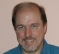For this I would use a "zero-phase" smoother (such as a moving average symmetric around its midpoint) on the COMPLEX spectrum, and then form the magnitude.

Note that doing this effectively shortens your "window" in the time domain.  For example, the spectral-smoother (1/4, 1/2, 1/4) corresponds to a zero-phase Hann window in the time domain (when there was no zero-padding).

[ - ]Thanks!

I will try to follow the suggestions so far for the implementation.

It would be helpful if I could know any book or a paper that I can refer for more details.

[ - ]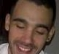What do you mean with "smooth"?

In case you want to eliminate the upper-band of the spectrum, filtering the signal in the time domain should be enough.

But in case you want to eliminate random noise to get a "better" spectrum of the speech signal, maybe you should take not a simple fft approach but a spectral estimation approach.

Depending on how much available data you have, there are many different approaches for the estimation of the speech signal spectrum. (e.g. Welch's Method, Blackman-Tukey Method, etc.)

[ - ]